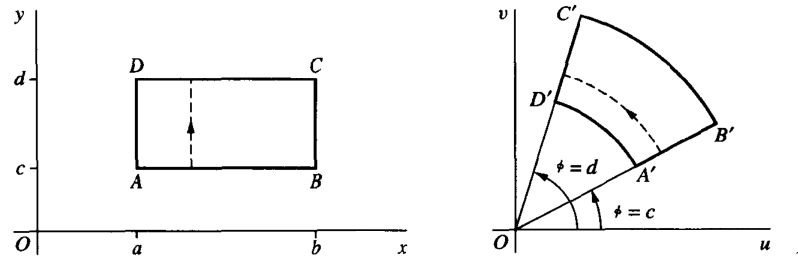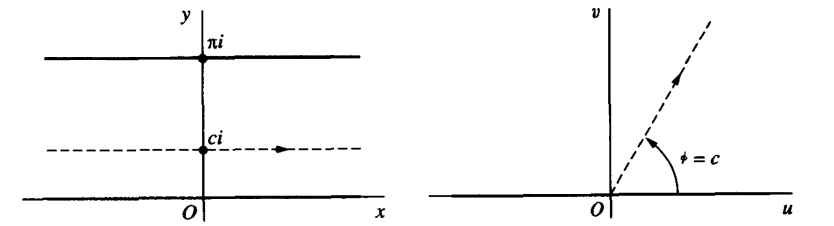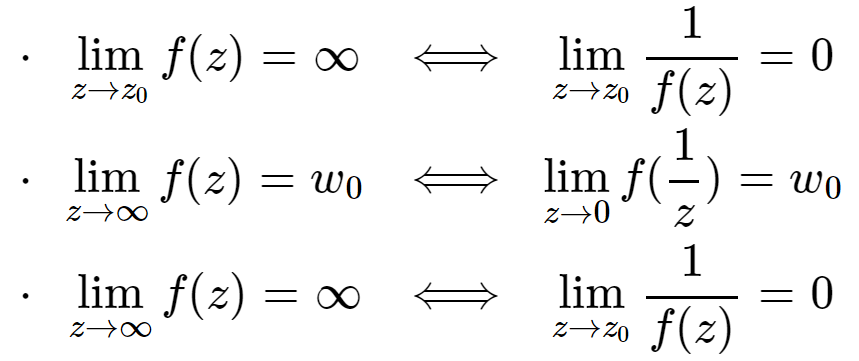• 复变函数复变函数复变函数复变函数复变函数复变函数复变函数复变函数复变函数复变函数复变函数复变函数复变函数复变函数复变函数复变函数复变函数复变函数复变函数复变函数
• 复变函数复变函数复变函数复变函数复变函数复变函数复变函数复变函数复变函数复变函数复变函数复变函数
• 复变函数复变函数复变函数复变函数复变函数复变函数 复变函数复变函数
• 以复数作为自变量和因变量的函数就叫做复变函数，而与之相关的理论就是复变函数论。解析函数是复变函数中一类具有解析性质的函数，复变函数论主要就研究复数域上的解析函数，因此通常也称复变函数论为解析函数论。 ...
        以复数作为自变量和因变量的函数就叫做复变函数，而与之相关的理论就是复变函数论。解析函数是复变函数中一类具有解析性质的函数，复变函数论主要就研究复数域上的解析函数，因此通常也称复变函数论为解析函数论。
复数的概念起源于求方程的根，在二次、三次代数方程的求根中就出现了负数开平方的情况。在很长时间里，人们对这类数不能理解。但随着数学的发展，这类数的重要性就日益显现出来。

复变函数论产生于十八世纪。1774年， 欧拉在他的一篇论文中考虑了由复变函数的积分导出的两个方程。而比他更早时，法国数学家 达朗贝尔在他的关于流体力学的论文中，就已经得到了它们。因此，后来人们提到这两个方程，把它们叫做“达朗贝尔- 欧拉方程”。到了十九世纪，上述两个方程在 柯西和 黎曼研究流体力学时，作了更详细的研究，所以这两个方程也被叫做“柯西-黎曼条件”。

复变函数论的全面发展是在十九世纪，就像 微积分的直接扩展统治了十八世纪的数学那样，复变函数这个新的分支统治了十九世纪的数学。当时的数学家公认复变函数论是最丰饶的数学分支，并且称为这个世纪的数学享受，也有人称赞它是抽象科学中最和谐的理论之一。

为复变函数论的创建做了最早期工作的是欧拉、达朗贝尔，法国的 拉普拉斯也随后研究过复变函数的积分，他们都是创建这门学科的先驱。

后来为这门学科的发展作了大量奠基工作的要算是柯西、黎曼和德国数学家 维尔斯特拉斯了。二十世纪初，复变函数论又有了很大的进展，维尔斯特拉斯的学生， 瑞典数学家列夫勒、法国数学家 庞加莱、 阿达玛等都作了大量的研究工作，开拓了复变函数论更广阔的研究领域，为这门学科的发展做出了贡献。

复变函数论在应用方面，涉及的面很广，有很多复杂的计算都是用它来解决的。比如物理学上有很多不同的稳定平面场，所谓 场就是每点对应有 物理量的一个区域，对它们的计算就是通过复变函数来解决的。

比如俄国的茹柯夫斯基在设计飞机的时候，就用复变函数论解决了飞机机翼的结构问题，他在运用复变函数论解决流体力学和航空力学方面的问题上也做出了贡献。

复变函数论不但在其他学科得到了广泛的应用，而且在数学领域的许多分支也都应用了它的理论。它已经深入到微分方程、 积分方程、 概率论和 数论等学科，对它们的发展很有影响。

展开全文• 复变函数习题选解 复变函数习题选解 复变函数习题选解 复变函数习题选解 复变函数习题选解 复变函数习题选解
• 复变函数复变函数复变函数复变函数复变函数复变函数复变函数复变函数
• 复变函数与积分变换 复变函数与积分变换 复变函数与积分变换
• 6.1复数 6.1.1复数的表示 6.1.2复数的常用命令 6.1.3复数的生成和基本运算 6.1.4复数方程求解 6.2复变函数的极限 6.3复变函数的导数 6.4复变函数的定积分 6.5复变函数的级数 6.6复变函数的泰勒展开 6.7留数计算 ...
• 复变函数习题详解及导学复变函数习题详解及导学复变函数习题详解及导学复变函数习题详解及导学复变函数习题详解及导学复变函数习题详解及导学复变函数习题详解及导学复变函数习题详解及导学复复变函数习题详解及导学...
• 这个总结文章本来是学完复变函数之后的复习总结，打印应付考试用的，后来假期里面又添加了一些公式、注意点什么的，稍稍完善了一些。 本文主要整理自我的复变函数老师的课件和作业、相关教材和上课笔记，不做商用，...
这个总结文章本来是学完复变函数之后的复习总结，打印应付考试用的，后来假期里面又添加了一些公式、注意点什么的，稍稍完善了一些。
本文主要整理自我的复变函数老师的课件和作业、相关教材和上课笔记，不做商用，侵删。
一方面考虑到当初我学习的时候四处查资料的痛苦，就想服务一下学习复变的孩子们，另一方面也想整理一个合集，方便后面课程学习时查找（比如电磁波），还有就是想要鞭策自己啦，别再咕咕咕了。
手打公式难免有些小问题，如果有什么错误欢迎大家指正哈，评论或者私信都可以。
这一篇包含复变函数的主要内容，包括：

复数及其运算，复变函数及其性质
解析函数，导数
柯西积分定理，柯西积分公式
幂级数和泰勒级数
洛朗级数
留数定理

一、复变函数
复数和复变函数
复数及运算
复数的表示：

$z=x+iy$
$z=\rho (\cos \varphi +i \sin \varphi)$
$\displaystyle z=\rho e^{i\varphi}$

$\rho$是复数的模，$\varphi=Arg z=arg z+2k\pi$是复数的辐角。
共轭复数：$\displaystyle z^{*}=x-iy=\rho e^{-i\varphi}$
复数的运算：

加法
$z_1+z_2=(x_1+x_2)+i(y_1+y_2)$
有三角不等式：
$|z_1+z_2| \leq |z_1| +|z_2|$

减法
$z_1-z_2=(x_1-x_2)+i(y_1-y_2)$
有三角不等式：
$|z_1-z_2|\geq|z_1|-|z_2|$

乘法
$z_1z_2=(x_1x_2-y_1y_2)+i(x_1y_2+x_2y_1)$
$z_1z_2=\rho_1 \rho_2 e^{i(\varphi_1+\varphi_2)}$

除法
$\frac{z_1}{z_2}=\frac{x_1+iy_1}{x_2+iy_2}=\frac{x_1x_2+y_1y_2}{x_2^2+y_2^2}+i\frac{x_2y_1-x_1y_2}{x_2^2+y_2^2}$
$\frac{z_1}{z_2}=\frac{\rho_1}{\rho_2}e^{i(\varphi_1-\varphi_2)}$

乘方
$z^n=\rho^n e^{in\varphi}$

开方
$\sqrt[n]{z}=\sqrt[n]{\rho}e^{i\frac{\varphi}{n}}$

注：在计算复数的开方时，要注意将辐角$\varphi$写成$argz+2k\pi$的形式，便于确定最终开方后得到的复数的个数。
复变函数及性质

几个常见的初等复变函数：

\begin{aligned} & e^z=e^{x+iy}=e^x(\cos y+i \sin y)\\ & \sin z=\frac{1}{2i}(e^{iz}-e^{-iz}), \cos z=\frac{1}{2}(e^{iz}+e^{-iz})\\ & sh z=\frac{1}{2}(e^z-e^{-z}), ch z=\frac{1}{2}(e^z+e^{-z})\\ & \ln z=\ln (|z|e^{i Arg z})=\ln |z|+ iArg z\\ & z^s=e^{s \ln z} \end{aligned}

复变函数$f(z)=u(x,y)+iv(x,y)$可以归结为对应于一对二元实变函数。

解析函数
复变函数导数

复变函数可导的定义：

函数$w=f(z)$是在区域B上定义的单值函数，在B上某点z，极限
$\lim_{\Delta z \to 0}{\frac{\Delta w}{\Delta z}}=\lim_{\Delta z \to 0}{\frac{f(z+\Delta z)-f(z)}{\Delta z}}$
存在且与$\Delta z\to 0$的方式无关，则称函数$w=f(z)$在z点可导。

复变函数的导数满足实变函数导数中的大多数运算性质，如加法、减法、乘法、除法、求倒、链式法则。

函数可导的必要条件：Cauchy-Riemann方程/条件

\begin{aligned} \frac{\partial u}{\partial x}& =\frac{\partial v}{\partial y}\\ \frac{\partial v}{\partial x}&=-\frac{\partial u}{\partial y} \end{aligned}
此时：
$f'(z)=\frac{\partial u}{\partial x}+i\frac{\partial v}{\partial y}$

函数可导的充要条件: u(x,y),v(x,y)都可微，且满足柯西-黎曼条件。

解析函数

定义

如果f(z)在点$z_0$及其邻域上处处可导，则称f(z)在点$z_0$解析；
如果f(z)在区域B上的每一点解析，则称f(z)是区域B上的解析函数。

**若函数f(z)=u+iv在区域B上解析，则u,v都是区域B上的调和函数。**即：

$\frac{\partial^2 u}{\partial x^2}+\frac{\partial ^2 u}{\partial y^2}=0, \frac{\partial ^2 v}{\partial x^2}+\frac{\partial ^2 v}{\partial y^2}=0$

计算$\displaystyle v=\int dv$的方法：

曲线积分法：选取特殊路径（比如：矩形的两边）作为积分路径；
凑全微分显式法；
不定积分法：对每个变量逐个积分。

函数解析的充要条件：u,v在区域B上可微，且满足柯西-黎曼条件。

注：

解析函数的实部或虚部必须是调和函数；
求$\displaystyle v=\int dv$时，可以采用极坐标。

复变函数积分
复变函数积分
对$z=x+iy,f=u+iv$，
$\int_l f(z) dz=\int _l (udx-vdy)+i\int_l(vdx+udy)$
柯西积分定理

单连通区域柯西定理

如果函数f(z)在闭单连通区域$\overline{B}$上解析，则沿$\overline{B}$上任何一段光滑闭合曲线$l$（也可以是$\overline{B}$的边界），有：
$\oint_l f(z)dz=0$

复连通区域柯西定理

如果函数f(z)是闭复连通区域$\overline{B}$中的单值解析函数，则有：
$\oint_l{f(z)dz}+\sum_{i=1}^{n}{\oint_{l_i}f(z)dz}=0$
其中，$l$表示区域外边界线，$l_i$表示区域内边界线，积分均按照边界线的正方向进行。
复连通区域柯西定理也可以写作下列形式：（更加常用的形式，在边界里面挖洞之后将外边界积分转化为内边界积分之和）
$\oint_l f(z)dz=\sum_{i=1}^n \oint_{l_i}{f(z)dz}$
积分均按照逆时针方向。

非常有用的一个环路积分(尤其是在留数定理中应用甚广)

\begin{aligned} & \frac{1}{2\pi i}\oint_l{\frac{dz}{z-\alpha}}= \left\{ \begin{array}{rcl} & 0 & for & l不包围\alpha\\ &1 & for & l包围\alpha \end{array} \right .\\ & \frac{1}{2\pi i }\oint_l{(z-\alpha)^ndz}=0, (n \neq -1) \end{aligned}
柯西积分公式

柯西积分公式

$f(\alpha)=\frac{1}{2\pi i}\oint_l {\frac{f(\zeta)}{\zeta -z}d\zeta}$
$f^{(n)}(z)=\frac{n!}{2\pi i}\oint_l{\frac{f(\zeta)}{(\zeta -z)^{n+1}}d\zeta}$
利用柯西积分公式可以将积分转化为某点的函数值。

刘维尔定理

如果函数f(z)在全平面上解析，并且是有界的，即$|f(z)\leq M|$，则函数f(z)一定为常数。
幂级数和泰勒级数

有复数项的无穷级数的收敛问题，可以转化为实部和虚部两个实数项级数的收敛问题。

幂级数
对幂级数$\displaystyle \sum_{k=0}^{\infty}{a_k(z-z_0)^k}$，引入记号
$R=\lim_{k\to\infty}\vert \frac{a_k}{a_{k+1}} \vert$
若$|z-z_0|，则幂级数收敛。
幂级数在收敛圆的内部（比半径为R的圆稍小一点的闭区域）收敛，在收敛圆外部发散。
幂级数逐项积分和逐项微分都不改变收敛半径。
泰勒级数

定义：

设f(z)在以$z_0$为圆心的圆$C_R$上解析，则对圆内的任意z点，f(z)都可以展成幂级数：
$f(z)=\sum_{k=0}^{\infty}a_k(z-z_0)^k,$
其中，
$a_k=\frac{1}{2\pi i}\oint_{C_{R_1}}\frac{f(\zeta)}{(\zeta-z_0)^{k+1}}d\zeta=\frac{1}{k!}f^{(k)}(z_0)$
$C_{R_1}$是$C_R$圆内包含z且与$C_R$同心的圆。

常用的泰勒级数展开

\begin{aligned} & \frac{1}{1-z}=1+z+z^2+\dots, |z|<1\\ & e^z=\sum_{k=0}^{\infty}{\frac{z^k}{k!}}\\ & \sin z=\sum _{k=0}^{\infty}{\frac{(-1)^k}{(2k+1)!}z^{2k+1}}\\ & \cos z=\sum_{k=0}^{\infty}{\frac{(-1)^k}{(2k)!}z^{2k}} \end{aligned}

注：求某个函数的泰勒展开时，可以考虑用一些基本函数的泰勒展开代入、求导、积分等转化。

洛朗级数
洛朗级数
定义：
设f(z)在环形区域$R_2<|z-z_0|内部单值解析，则对于环域上任何一点z，f(z)可以展开为双边幂级数：
$f(z)=\sum_{k=-\infty}^{\infty}{a_k(z-z_0)^k}$
其中，
$a_k=\frac{1}{2\pi i}\oint_l{\frac{f(\zeta)}{(\zeta-z_0)^{k+1}}d\zeta}$
这里，$l$是环域内的绕内圆一周的任意闭合曲线。

将一个函数展开为洛朗级数，一般会用到下面的式子：
$\displaystyle \frac{1}{1-z}=\sum_{k=0}^{\infty}z^k,|z|<1$

孤立奇点的分类和判定

孤立奇点的定义：f(z)在$z_0$点不解析，但在$z_0$的$0<|z-z_0|<\delta$内解析，即在$z_0$的领域内解析。

孤立奇点的分类和判定：
$\Zeta=\lim_{z\to z_0}f(z)$

类型
定义
判定
取舍

可去奇点
展开式中不含有$z-z_0$的负幂项
$\Zeta=c_0$为常数
不作为奇点看待

极点
展开式中含有有限项$z-z_0$的负幂项
$\Zeta=\infty$
留数可求且为重点

本性奇点
展开式中含有无限项$z-z_0$的负幂项
$\Zeta$不存在且不为$\infty$
难以刻画

零点和极点的关系：$z_0$是f(z)的m级零点$\Leftrightarrow$$z_0$是$\displaystyle \frac{1}{f(z)}$的m级极点。

留数定理

留数的定义

$z_0$是$f(z)$的孤立奇点，即存在R使$f(z)$在圆环$0<|z-z_0|内解析，其洛朗展开为：
$f(z)=\sum_{k=-\infty}^{\infty}{a_k(z-z_0)^k}$
其中项$(z-z_0)^{-1}$的系数
$a_{-1}=\frac{1}{2\pi i}\oint_{|z-z_0|=r}f(z)dz,0
称为f(z)在点$z_0$的留数，记作$Res f(z_0)$

留数定理

设函数f(z)在回路$l$上所围区域B上除有限个孤立奇点$z_1,z_2,\dots,z_n$外解析，在闭区域$\overline{B}$上除$z_1,z_2,\dots,z_n$外连续，则：
$\oint_l f(z)dz=2\pi i \sum_{k=1}^{n}Res f(z_k)$

留数定理将积分问题转化为留数求解问题。

留数求解

单极点

\begin{aligned} & Res f(z_0)=\lim_{z\to z_0}((z-z_0)f(z));\\ & Res f(z_0)=\lim_{z\to z_0}\frac{(z-z_0)P(z)}{Q(z)}=\frac{P(z_0)}{Q'(z_0)}，对于f(z)=\frac{P(z)}{Q(z)}且P(z_0)\neq 0,z_0是Q(z)的一阶零点。\\ & Res f(z_0)=\frac{(z+1)P^{(n)}(z_0)}{Q^{(n+1)}(z_0)}，对于f(z)=\frac{P(z)}{Q(z)}且z_0是Q(z)的n+1阶零点，又是P(z)的n阶零点。 \end{aligned}

n阶极点

$Res f(z_0)=\lim_{z\to z_0}\frac{1}{(z-1)!}(\frac{d^{n-1}}{dz^{n-1}}((z-z_0)^nf(z)))$

∞的留数

若∞是f(z)的孤立奇点，即存在$R>0$使得f(z)在圆环$R<|z-z_0|<+\infty$内解析，其洛朗展开为：
$f(z)=\sum_{k=-\infty}^{\infty}a_kz^k$
其中$z^{-1}$项的系数的相反数被称为∞点的留数，即：
$Res f(\infty)=-a_{-1}=\frac{1}{2\pi i}\oint_{|z|=r}f(z)dz, r
可以证明：函数f(z)若在扩充复平面上只有有限个孤立奇点，则f(z)在各点的留数之和等于0。即：
$Res f(\infty)+\sum_{k=1}^{n}Res f(z_k)=0$

留数定理在积分问题中的应用

类型一
$I=\int_0^{2\pi}R(\cos x,\sin x)dx$
被积函数是三角函数的有理式，积分区间是$[0,2\pi]$。
方法是变量替换：
\begin{aligned} & z=e^{ix}\\ \therefore & \cos x=\frac{1}{2}(z+z^{-1}),\sin x=\frac{1}{2i}(z-z^{-1}),dx=\frac{1}{iz}dz \end{aligned}
注意不要用共轭复数$z^*$，注意积分空间是在单位圆内从而确定在单位圆内的极点个数

类型二
$I=\int_{-\infty}^{\infty}f(x)dx$
积分空间为$(-\infty, \infty)$，复变函数f(z)在实轴上没有奇点，在上半平面除了有限个奇点外都是解析的；当z在上半平面及实轴上$\to \infty$时，zf(z)一致的$\to \infty$。
$I=\int_{-\infty}^{\infty}f(x)dx=2\pi i\sum_{z_0\in\{上半平面内奇点全体\}}Res f(z_0)$

类型三
\begin{aligned} & I_1=\int_0^{\infty}f(x)\cos mx dx\\ & I_2=\int_0^{\infty}g(x)\sin mx dx \end{aligned}
积分区间$[0,+\infty)$，偶函数f(x)和奇函数g(x)在实轴上没有奇点，在上半平面除了有限个奇点外都是解析的；当z在上半平面及实轴上$\to \infty$时，f(z)和g(z)一致的$\to \infty$。
当m>0时：
\begin{aligned} & I_1=\int_0^{\infty}f(x)\cos mx dx=\frac{1}{2}\int_{-\infty}^{\infty}f(x)e^{imx}dx=\pi i\sum_{z_0\in\{上半平面内全体奇点\}}Res (f(z_0)e^{imz_0})\\ & I_2=\int_0^{\infty}g(x)\sin mx dx=\frac{1}{2i}\int_{-\infty}^{\infty}g(x)e^{imx}dx=\pi \sum_{z_0\in\{上半平面内全体奇点\}}Res(g(z_0)e^{imz_0}) \end{aligned}
当m<0时：
\begin{aligned} & I_1=\int_0^{\infty}f(x)\cos mx dx=-\pi i\sum_{z_0\in\{下半平面内全体奇点\}}Res (f(z_0)e^{imz_0})\\ & I_2=\int_0^{\infty}g(x)\sin mx dx=-\pi \sum_{z_0\in\{下半平面内全体奇点\}}Res(g(z_0)e^{imz_0}) \end{aligned}

类型四
$I=\int_{-\infty}^{\infty}f(x)dx$
函数f(z)在实轴上有单极点$z=\alpha$，除此之外，函数f(z)满足类型二或者类型三。
$\int_{-\infty}^{\infty}f(x)dx=2\pi i\sum_{z_0\in\{上半平面内全体奇点\}}Res f(z_0)+\pi i \sum_{\alpha \in \{实轴上的全体奇点\}}Res f(\alpha)$
α只能是单极点，当为二阶及以上极点时，积分为∞，当为本性奇点时，积分不存在。


展开全文数学
• 复变函数和积分变换复变函数和积分变换复变函数和积分变换复变函数和积分变换复变函数和积分变换复变函数和积分变换复变函数和积分变换复变函数和积分变换复变函数和积分变换
•matlab matlab安装教程 matlab下载 matlab图像处理
• 复变函数与积分变换复变函数与积分变换复变函数与积分变换复变函数与积分变换复变函数与积分变换复变函数与积分变换
• 复变函数 复变函数定义 对应实变函数，同样也可以建立从复变量映射到复变量的函数。 即可以定义映射 f:S↦S∗f:S\mapsto S^*f:S↦S∗，S,S∗⊆CS,S^*\subseteq\mathbb{C}S,S∗⊆C，对于任一复数 z∈Sz\in Sz∈S，都...
文章目录2.1$\quad$复变函数2.1.1$\quad$复变函数的定义2.1.2$\quad$复变函数的图像表示2.1.3$\quad$复变函数在不同坐标下的形式转换2.1.4$\quad$复变函数的反函数2.2$\quad$复变函数的极限和连续性2.2.1$\quad$复变函数的极限$\bold{Ⅰ}.\ \,$极限的定义$\bold{Ⅱ}.\ \,$极限定理$\bold{Ⅲ}.\ \,$无穷远点的极限2.2.2$\quad$复变函数的连续性$\bold{Ⅰ}.\ \,$连续的定义$\bold{Ⅱ}.\ \,$连续性定理2.3$\quad$复变函数的导数和微分2.3.1$\quad$复变函数的导数$\bold{Ⅰ}.\ \,$导数的定义$\bold{Ⅱ}.\ \,$导数的性质2.3.2$\quad$复变函数的微分2.4$\quad$解析函数2.4.1$\quad$解析函数的定义2.4.2$\quad$柯西-黎曼方程$\bold{Ⅰ}.\ \,$解析的充要条件$\bold{Ⅱ}.\ \,$解析函数的性质
2.1$\quad$复变函数
2.1.1$\quad$复变函数的定义
对应实变函数，同样也可以建立从复变量映射到复变量的函数。
即可以定义映射 $f:S\mapsto S^*$，$S,S^*\subseteq\mathbb{C}$，对于任一复数 $z\in S$，都对应一个或多个 $w\in S^*$. 用记号表示为 $w=f(z)$.
上述表述中，$S$ 为 定义集合，$S^*$ 为 函数值集合；$S$ 是 $f$ 的 定义域；$w$ 是 $z$ 的 象（映象），$z$ 是 $w$ 的 原象 。
为了形象地表示复变函数的映射情况，通常用从一个复平面映射到另一个复平面的方式来体现函数的几何特征。

2.1.2$\quad$复变函数的图像表示
【例 $2\verb|-|1$】用图像表示函数 $w=z^2$.具体变换式与曲线对应方程如下：第一组方程描述 $x\verb|-|y$ 平面映射到 $u\verb|-|v$ 平面的变换。
第二、三组方程描述 $u\verb|-|v$ 平面上平行于 $x,y$ 两轴的直线对应在 $x\verb|-|y$ 平面中的原象。
当然也可以观察辐角的变化：同时也可以观察 $u\verb|-|v$ 平面上的每个单位小正方形区域对应在 $x\verb|-|y$ 平面中的原象：不难看出，在两复平面内，原象中的两条相交直线形成的夹角在经过变换后没有发生变化。称满足此条件的函数映射本身为 共形映射 或 保形映射 。共形映射和的具体内容会在之后提及。

2.1.3$\quad$复变函数在不同坐标下的形式转换
同一个函数在不同类型的平面内几何特征也不同。上例中若将 $x\verb|-|y$ 平面与 $u\verb|-|v$ 平面都转化为极坐标，可得到下面的图像：容易看出，该例中极坐标下函数的图像变得尤其简单。转化坐标对研究某些函数很有帮助。
同样地，也可以将复变函数用指数函数形式来表示。

【例 $2\verb|-|2$】用图像表示函数 $w=e^z$.
以下直接给出图像的两复平面上的等值线与辐角的对应关系图像。2.1.4$\quad$复变函数的反函数
复变函数也有反函数的概念。按照实变函数的定义进行类比，可以得到如下定义：
若函数 $w=f(z)$ 的定义集合为 $z$ 平面上的集合 $S$，函数值集合为 $w$ 平面上的集合 $S^*$。对于映射 $f:S\mapsto S^*$，$S^*$上每一点 $w$ 必对应着 $S$ 中的一个（或多个）点，由此在 $S^*$ 上就确定了一个单值（或多值）函数 $z=\varphi(w)$，称为函数 $w=f(z)$ 的 反函数，也称为映射 $w=f(z)$ 的 逆映射。
也即：对于任意的 $w\in S^*$，有$w=f[\varphi(w)],$当反函数为单值函数时，也有$z=\varphi[f(z)],\quad z\in G.$
称正映射与逆映射都为单值的函数是 一一的，称对应的集合 $S$ 和 $S^*$ 是 一一对应 的。

2.2$\quad$复变函数的极限和连续性
2.2.1$\quad$复变函数的极限
$\bold{Ⅰ}.\ \,$极限的定义
设函数 $f$ 在$z_0$ 的去心邻域中有定义，当 $z$ 趋近于 $z_0$ 时，$\lim_{z\to z_0}{f(z)}=w_0$指 $w=f(z)$ 可以无限接近于 $w_0$，只要 $z$ 足够接近 $z_0$ 而不等于它。
按照实变函数中极限的 $\varepsilon\verb|-|\delta$ 定义，即：对于 $\forall\ \varepsilon>0$，$\exist\ \delta>0$，$\text{s.t.}$ $\ \ \ 0<|z-z_0|<\delta$ 时，$|f(z)-w_0|<\varepsilon.$
注意：极限定义中 $z$ 趋向于 $z_0$ 的方式是任意的。（类比多元实变函数极限）

$\bold{Ⅱ}.\ \,$极限定理
定理一  【极限唯一定理】$\quad$设函数 $f(z)$ 在一点 $z_0$ 处存在极限值，那么极限值唯一。
定理二  【极限分部定理】$\quad$设函数 $f(z)=u(x,y)+iv(x,y),\quad A=u_0+iv_0,\quad z_0=x_0+iy_0,$ 那么$\displaystyle{\lim_{z\to z_0}{f(z)}=A}$ 的充要条件是 $\lim_{(x,y)\to(x_0,y_0)}{u(x,y)}=u_0,\quad\lim_{(x,y)\to(x_0,y_0)}{v(x,y)}=v_0​.$
定理三  【极限四则运算】$\quad$设 $\displaystyle{\lim_{z\to z_0}{f(z)=A}},\,\,\displaystyle{\lim_{z\to z_0}{g(z)=B}}$，那么
$\qquad\qquad\qquad\qquad\qquad\ \, 1. \ \displaystyle{\lim_{z\to z_0}{f(z)\pm g(z)=A\pm B}\; ;}$
$\qquad\qquad\qquad\qquad\qquad\ \,2. \ \displaystyle{\lim_{z\to z_0}{f(z)g(z)=AB}\; ;}$
$\qquad\qquad\qquad\qquad\qquad\ \,3. \ \displaystyle{\lim_{z\to z_0}{\frac{f(z)}{g(z)}=\frac{A}{B}}\quad (B\ne 0).}$
$\bold{Ⅲ}.\ \,$无穷远点的极限
将无穷远点 $\infty$ 归入复平面，并且使用相关的极限能够带来许多便利。将纳入了无穷远点的复平面称为 扩充复平面 。
在扩充复平面上表示无穷远点时，可以先想象在复平面原点的正上方存在一个球与之相切于原点。定义球的北极 $N$ 为球上在原点正上方的点，南极 $S$ 即为原点 $O$ ，则原来的复平面上任一点 $z$ 都能与 $N$ 相连并交于球面与另一点 $P$ ，如图所示。由此，我们可以建立 $P$ 与 $z$ 的一一对应关系。而北极点 $N$ 则定义为无穷远点。上述定义的球面被称为 复球面 或 黎曼球面。它是一种对复数平面加上一个无穷远点的扩张。引入无穷远点以后，我们可以在原有极限的定义中，把 $z$ 或 $w$ 的适当的领域替换为 $\infty$ ，并且以下定理成立：上述定理均可用 $\varepsilon\verb|-|\delta$ 定义证明。

2.2.2$\quad$复变函数的连续性
$\bold{Ⅰ}.\ \,$连续的定义
类比实变函数，复变函数的连续性定义也以极限的概念为基础。
复变函数的连续性定义由以下三个条件共同决定：

$f(z_0)$ 存在 $;$
$\displaystyle{\lim_{z\to z_0}{f(z)}}$ 存在 $;$
$\displaystyle{\lim_{z\to z_0}{f(z)}=f(z_0)}$ $.$

当然，第三个条件也可以用极限的 $\varepsilon\verb|-|\delta$ 定义描述。
如果 $f(z)$ 在区域 $D$ 范围内处处连续，则称 $f(z)$ 在 $D$ 内连续。
$\bold{Ⅱ}.\ \,$连续性定理
定理一  【连续分部定理】$\quad$函数 $f(z)=u(x,y)+iv(x,y)$ 在 $z_0=x_0+iy_0$ 处连续的充要条件是：$u(x,y)$ 和 $v(x,y)$ 在 $(x_0,y_0)$ 处连续。
定理二  【连续四则运算】$\quad$在 $z_0$ 处连续的两个函数 $f(z),g(z)$ 的和、差、积、商（$z_0$ 分母不为 $0$） 在 $z_0$ 处仍连续。
定理三  【连续复合定理】$\quad$若函数 $h=g(z)$ 在 $z_0$ 处连续，函数 $w=f(h)$ 在 $h_0=g(z_0)$ 处连续，则复合函数 $w=f[g(z)]$在 $z_0$ 处连续。
定理三  【有界性定理】$\quad$在闭曲线或包括曲线在内的曲线段上连续的函数 $f(z)$ 在曲线上有界。即存在一正数 $M$，在曲线上恒有$|f(z)|\le M.$

2.3$\quad$复变函数的导数和微分
2.3.1$\quad$复变函数的导数
$\bold{Ⅰ}.\ \,$导数的定义
设函数 $w=f(z)$ 定义于区域 $D$，$z_0$为 $D$ 中的一点，点 $z_0+\Delta z$ 不出 $D$ 的范围。如果极限 $\displaystyle{\lim_{\Delta z\to 0}{\frac{f(z_0+\Delta z)-f(z_0)}{\Delta z}}}$ 存在，那么就说 $f(z)$ 在 $z_0$ 处 可导 ，这个极限值称为 $f(z)$ 在 $z_0$ 的 导数，记作：$f'(z_0)=\frac{dw}{dz}|_{z=z_0}=\lim_{\Delta z\to 0}{\frac{f(z_0+\Delta z)-f(z_0)}{\Delta z}}.$
注意：导数定义中 $z_0+\Delta z\to z_0$（即$\Delta z\to 0$）的方式是任意的，所以复变函数对于导数的限制比对一元实变函数的类似限制要严格很多，并且是复变可导函数有了独特的性质和应用。
若 $f(z)$ 在区域 $D$ 内处处可导，则称 $f(z)$ 在 $D$ 内可导 。
$\bold{Ⅱ}.\ \,$导数的性质

四则运算

$(c)'=0$
$(z^n)'=nz^{n-1}$
$[f(z)\pm g(z)]'=f'(z)\pm g'(z)$
$[f(z)g(z)]'=f'(z)g(z)+f(z)g'(z)$
$\displaystyle{[\frac{f(z)}{g(z)}]'=\frac{1}{g^2(z)}[g(z)f'(z)-f(z)g'(z)]}$
${f[g(z)]}'=f'[g(z)]g'(z)$
$\displaystyle{f'(z)=\frac{1}{\varphi '(w)}}\quad(w=f(z)$ 与 $z=\varphi(w)$ 互为反函数$)$

性质
可导一定连续，连续不一定可导。

2.3.2$\quad$复变函数的微分
复变函数的微分概念在形式上和实变函数的微分概念完全相同。
设函数 $w=f(z)$ 在 $z_0$ 处可导，则 $\Delta w=f(z_0+\Delta z)-f(z_0)=f'(z_0)\Delta z+\rho(\Delta z)\Delta z.$其中$\displaystyle{\lim_{\Delta z\to 0}{\rho(\Delta z)}=0.}$ 因此，$|\rho(\Delta z)\Delta z|$ 是 $\Delta z$ 的高阶无穷小量，而 $f'(z_0)\Delta z$ 是函数 $w=f(z)$ 的线性部分，称为函数 $w=f(z)$ 在点 $z_0$ 处的微分，记作：$dw=f'(z_0)dz,$即$f'(z_0)=\frac{dw}{dz}|_{z=z_0}.$
若 $f(z)$ 在区域 $D$ 内处处可微，则称 $f(z)$ 在 $D$ 内可微 。
从上述过程中可以知道，函数 $w=f(z)$ 在 $z_0$ 处可导与在 $z_0$ 处可微是等价的。

2.4$\quad$解析函数
2.4.1$\quad$解析函数的定义
复变函数中，解析的概念比可导更重要。
如果函数 $f(z)$ 在 $z_0$ 及其邻域内处处可导，则称 $f(z)$ 在 $z_0$ 解析 。而函数 $f(z)$ 在区域 $D$ 内每一点解析，则称 $f(z)$ 在 $D$ 内解析，或称 $f(z)$ 是 $D$ 内的一个 解析函数 。
如果 $f(z)$ 在 $z_0$ 处不解析，则称 $z_0$ 为 $f(z)$ 的 奇点 。
由定义我们就直接可以看出：函数在某点可导，不一定在该点解析（邻域不可导）；函数在某点解析，则一定在该点可导。
而根据求导法则，我们也可以得出：

在区域 $D$ 内解析的两个函数 $f(z),g(z)$ 的和、差、积、商（去除分母为 $0$ 的点） 在 $D$ 内仍解析。
若函数 $h=g(z)$ 在 $D$ 内解析，函数 $w=f(h)$ 在 $h)$ 平面上的区域 $G$ 解析。如果对 $D$ 内的每一个点 $z$，函数 $g(z)$ 的对应值 $h$ 都属于 $G$，则复合函数 $w=f[g(z)]$在 $D$ 内解析。

2.4.2$\quad$柯西-黎曼方程
$\bold{Ⅰ}.\ \,$解析的充要条件
与实变函数的全微分类似，复变函数对于一个函数是否解析只用定义判断是不够的。从导数的定义出发，我们可以得到更好的函数解析的充要条件——柯西-黎曼方程。
函数在某点处可导的充要条件$\quad$设函数 $f(z)=u(x,y)+iv(x,y)$ 定义在区域 $D$ 内，则 $f(z)$ 在 $D$ 内一点 $z=x+iy$ 可导的充要条件为：$u(x,y)$ 与 $v(x,y)$ 在点 $(x,y)$ 可微，并且在该点满足 柯西-黎曼方程$\frac{\partial u}{\partial x}=\frac{\partial v}{\partial y},\qquad\frac{\partial u}{\partial y}=-\frac{\partial v}{\partial x}.$
函数解析的充要条件$\quad$函数 $f(z)=u(x,y)+iv(x,y)$ 在其定义域 $D$ 内解析的充要条件为：$u(x,y)$ 与 $v(x,y)$ 在 $D$ 内可微，并且满足柯西-黎曼方程。
同时，我们也可以得到可导函数 $f(z)=u(x,y)+iv(x,y)$ 在点 $z=x+iy$ 处的导数公式：$f'(z)=\frac{\partial u}{\partial x}+i\frac{\partial v}{\partial x}=\frac{1}{i}\frac{\partial u}{\partial y}+\frac{\partial v}{\partial y}.$
当然，上述的讨论只是在直角坐标下的。极坐标下对于函数解析可以利用上述结论，用链式法则推出。
函数在某点处可导的充要条件（极坐标下）$\quad$设函数 $f(z)=u(r,\theta)+iv(r,\theta)$ 定义在区域 $D$ 内，则 $f(z)$ 在 $D$ 内一点 $z=re^{i\theta}\ (z\ne 0)$ 可导的充要条件为：$u(r,\theta)$ 与 $v(r,\theta)$ 在点 $(r,\theta)$ 可微，并且在该点满足 柯西-黎曼方程$r\frac{\partial u}{\partial r}=\frac{\partial v}{\partial \theta},\qquad \frac{\partial u}{\partial \theta}=-r\frac{\partial v}{\partial r}.$
此时 $f'(z)$ 可以写为$f'(z)=e^{-i\theta}(\frac{\partial u}{\partial r}+i\frac{\partial v}{\partial r}).$
解析函数有许多好的性质，所以也相当重要。之后要学习的初等函数都是在整个复平面上解析的函数。我们把这样的函数称为 整函数 。
$\bold{Ⅱ}.\ \,$解析函数的性质
以上的内容能够更快速地对函数是否解析作出判别。而知道了一个函数是解析函数后，也能带来一些好的性质。

如果 $f(z)$ 在区域 $D$ 是解析的，且在区域 $D$ 内处处满足 $f'(z)=0$，则 $f(z)$ 在区域 $D$ 内为一常数。
如果 $f(z)=u+iv$ 为一解析函数，且 $f'(z)\ne 0$，那么曲线组 $u(x,y)=c_1$ 和 $v(x,y)=c_2$ 必互相正交，其中 $c_1,c_2$ 为常数。

第二条性质在上一部分中有所提及。由此可猜测，解析函数对应的映射属于共形映射。而此性质的确成立。


展开全文• 复变函数（2）-复变函数及其解析性                            东风夜放花千树，更吹落，星如雨       2.1 复变函数的定义：  设DDD是复平面上一个非空点集。如果...
复变函数（2）-复变函数及其解析性

东风夜放花千树，更吹落，星如雨

2.1 复变函数的定义：
设$D$是复平面上一个非空点集。如果按照一个确定的法则$f$，对于$D$中的每一个点$z$，都有一个或多个复数$w$与之对应，则称复变数$w$是复变数$z$的函数，简称为复变函数，记为
$w=f(z)\ ,\quad z\in D$ 如果$z$的一个值对应于$w$的一个值，那么称函数$f(z)$是单值的，如果$z$的一个值对应着$w$的多个值，那么称函数$f(z)$是多值的。
复变函数也可以和两个二元实函数$u(x,y)\ ,\ v(x,y)$联系起来，设$w=u+vi$，$z=x+yi$，则
$w=u+vi=f(z)=f(x+yi)=u(x,y)+v(x,y)i$ 复变函数可以类比实函数定义其函数特征。
2.2 指数函数：
对于复变数$z=x+yi$，称复变数$w=e^x(cosy+isiny)$为复变数$z$的指数函数，记作$w=e^z$，即
$w=e^z=e^x(cosy+isiny)$\left\{\begin{aligned} & |e^z|=e^x \\ & Arg(e^z)=y+2k\pi\quad (k=0,\pm1,\pm 2,\ldots)\end{aligned}\right. 指数函数是单值函数，且在复平面上处处有定义。
（注：这里单值函数是指在$z=x+yi$这样的实部虚部表示法下，函数值$w=e^z$有唯一确定的实部和虚部。对于虚数的指数表示法$Arg(e^z)=y+2k\pi$，在任何情况下根据定义辐角的取值都是无穷多的，所以不利用复变数的指数和三角表示法来判断函数的单值和多值性。）
复指数函数具有以下性质：
$e^0=1$$e^z\ne0$$e^{z_1+z_2}=e^z_1e^z_2$$e^{-z}=\frac{1}{e^z}$$\overline{e^z}=e^{\overline{z}}$$e^{2k\pi i}=1$
2.3 对数函数
指数函数的反函数，即满足方程
$e^w=z\quad (z\ne 0)$ 的复变数$w$称为复变数$z$的对数函数，记作$w=Ln\ z$。
对于$w=u+vi$
$|z|=|e^w|=e^u$$Arg\ z=Arg\ e^w=v$ 所以
$u=ln|z|\ ,\quad v=Arg\ z$$Ln\ z=ln|z|+iArg\ z\quad(z\ne 0)$$w=Ln\ z=ln|z|+iarg\ z+2k\pi i\quad (k=0,\pm1,\pm 2,\ldots)$ 可以看出对数函数可以得出多种实部和虚部表示，所以对数函数是多值函数。给定确定$k=k_0$时，可以得到对数函数的一个分支，对数函数的每一个分支都是单值函数。
$k=0$时的分支称为对数函数$w=Ln\ z$的主分支，对应的函数值为函数主值，记为$ln\ z$，有
$ln\ z=ln|z|+iarg\ z\quad (z\ne 0)$
对数函数有如下性质
$Ln(z_1z_2)=Ln\ z_1+Ln\ z_2$$Ln(\frac{z_1}{z_2})=Ln\ z_1-Ln\ z_2$ 等式两边都是多值函数，等号意味着两段可能取得的函数值全体相同。同理，下面的式子不成立
$Ln\ z^n\ne nLn\ z$$Ln\ \sqrt[n]{z}\ne \frac{1}{n}Ln\ z$
2.4 幂函数
设$a$是复常数，对于复变数$z\ne 0$，称复变数$w=e^{aLn\ z}$为复变数$z$的幂函数，即
$w=z^a=e^{aLn\ z}$ 当$a$为正实数，且$z=0$时，规定$z^a=0$。
指数函数的取值性需要分情况讨论。
(1)当$a=n$为整数时
$w=z^n=e^{nLn\ z}=e^{n[ln|z|+i(arg\ z+2k\pi)]}=e^{nln|z|+(narg\ z)i+2nk\pi i}=e^{nln|z|+(narg\ z)i}\quad (k=0,\pm1,\pm 2,\ldots)$ 所以此时幂函数为单值函数，当$n>0$时在复平面上处处有定义。当$n<0$时，在复平面除$z=0$点外处处有定义。
(2)当$a=\frac{p}{q}$（$p$，$q$为互质的整数，$q>0$）为有理数时
$w=z^a=e^{\frac{p}{q}Ln\ z}=e^{\frac{p}{q}[ln|z|+i(arg\ z+2k\pi)]}=e^{\frac{p}{q}ln|z|+(\frac{p}{q}arg\ z)i+2\frac{p}{q}k\pi i}$$=e^{\frac{p}{q}ln|z|}[cos\frac{p}{q}(arg\ z+2k\pi)+isin\frac{p}{q}(arg\ z+2k\pi))]\quad (k=0,\pm1,\pm 2,\ldots)$
根据三角函数的周期性，函数$w=z^{\frac{p}{q}}$具有$q$个不同的值，当$k=0,1,\ldots,q-1$时可以取得。
(3)当$a$为无理数或虚数时，幂函数是无穷多值的，在复平面上除了$z=0$外处处有定义，每一个单值分支对应于$Ln\ z$的一个单值分支。
2.5 三角函数
分别称
$\cos(z)=\frac{e^{iz}+e^{-iz}}{2}\quad \sin(z)=\frac{e^{iz}-e^{-iz}}{2i}$ 为复变数的余弦函数和正弦函数。
三角函数具有$2\pi$的周期性和奇偶性，但不再具有有界性，且
$\cos^2{z}+\sin^2z=1$$\cos(z+\frac{\pi}{2})=-\sin(z),\quad\cos(z+\pi)=-\cos z$$\sin(z+\frac{\pi}{2})=\cos(z),\quad\sin(z+\pi)=-\sin z$$\sin(z_1+z_2)=\sin z_1\cos z_2-\cos z_1\sin z_2$$\cos(z_1+z_2)=\cos z_1\cos z_2-\sin z_1\sin z_2$ 同理可以定义复变数的正切，余切，正割，余割函数。
$\tan z=\frac{\sin z}{\cos z},\quad\cot z=\frac{\cos z}{\sin z},\quad \sec z=\frac{1}{\cos z},\quad \csc z=\frac{1}{\sin z}$
2.6 反三角函数
余弦函数的反函数，满足$\cos w=z$的复变数$w$称为复变数$z$的反余弦函数，记作$w=Arc\cos z$